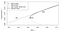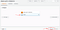# Deploying a Portfolio Investment Strategy in the Cloud

Two common portfolios investors use are the Maximum Sharpe Ratio (MSR) portfolio and the Global Minimum Variance (GMV) portfolio.

To construct the MSR portfolio, an investor needs to calculate the asset weights yielding the largest excess return per unit of risk (using the standard deviation of a portfolio’s return as the measure of risk). Constructing this portfolio requires two components: an estimate of the expected returns of each asset and an estimated covariance matrix, representing the covariance between each asset. In practice, it is difficult to calculate accurate estimates of the expected returns of assets.

The GMV portfolio is another portfolio on the efficient frontier. It yields returns with less volatility than an MSR portfolio. Investors need only to calculate a covariance matrix to construct this portfolio. The weights are independent of any expected returns. This is easier than constructing the MSR portfolio because it is not necessary to compute these estimated expected returns.MSR and GMV portfolios on the efficient frontier. Graph from https://arxiv.org/abs/1610.00937.

The GMV portfolio minimizes the variance of our portfolio. This means we select the portfolio weights that minimize the following formula. The formula for variance of a portfolio with N assets is

To implement the GMV portfolio in practice, I will be using Python, AWS Lambda, and Alpaca stock brokerage. We will construct a Python script that retrieves historical stock data, calculates daily returns, computes the covariance between returns, calculates the weights of the GMV portfolio, uses the Alpaca API to retrieve our current positions and portfolio value, and sends orders to Alpaca. We will have AWS Lambda run this function every weekday at 9:31AM. I will use a paper trading account, but this can be switched to a cash account by swapping out your paper API keys with your cash account API keys.

Let’s retrieve the stock tickers for the top 100 companies in the S&P 500, download daily OHLC data during the past year, and calculate the daily returns for each asset.

Define a function to calculate the weights of the GMV portfolio. For the estimated covariance, use the covariance of the daily asset returns over the past year. Run the function to calculate the asset weights in the GMV portfolio.

Authenticate with the Alpaca API. Store your API_KEY and API_SECRET as environment variables.

Cancel any open orders.

Calculate the target dollar amount to buy of each stock.

Define a function to submit orders to Alpaca.

Calculate the number of shares of each stock I need to buy or sell to meet the target dollar amounts. I’ll need to retrieve my current positions and construct orders that will take me from my current positions to my target positions.

Execute the sell orders first. Then execute the buy orders.

Let’s test out the script with a fresh Alpaca paper trading account. Test the following functionalities: 1.) Calculating and executing orders when the portfolio is 100% cash, and 2.) Calculating and executing orders when the portfolio already contains equities. Do this by calculating the GMV portfolio using an estimated covariance matrix based on returns from the past 5 years. Then run the script again to rebalance to a GMV portfolio based on a 10-year covariance matrix.

Run the script using 5-years of daily returns data and view the target dollar amounts for each asset:

We can verify from the out of the Python script that the orders were submitted:

We can also verify on the Alpaca Dashboard that the orders executed successfully

Now run the script again but use a covariance matrix calculated from 10 years of return data. These are the orders it creates:

This was only a minor rebalance because the covariance matrix was very similar, the price per share of the assets remained mostly the same (I ran the rebalance minutes later), and the portfolio value remained nearly the same.

Now that we’ve constructed the entire Python script and tested it, let’s have it run every weekday in AWS. Here’s a link to the full Python script I’ll be using that combines the above code snippets.

Create a deployment package containing this script along with the Python packages it uses that aren’t included in the AWS Lambda Python environment. We will utilize AWS Lambda Layers since our code base exceeds the 50MB zipped (or 250MB unzipped) threshold for Lambda functions. Layers allows us to reduce the size of our deployment package. We will use 3 layers. One for SciPy/NumPy, one for Pandas, and one for lxml. Create a new Lambda function with a Python 3.8 runtime. Click on Layers > Add a layer.Use AWS Layers to decrease the size of our deployment package

Use the layer provided by AWS that includes NumPy and SciPy.

For Pandas and lxml, we have to download the packages and create custom layers for them. Download this Pandas wheel file from PyPI: pandas-1.1.3-cp38-cp38-manylinux1_x86_64.whl. Pandas requires the pytz package, so download pytz-2020.1-py2.py3-none-any.whl from PyPI. Unzip the wheel files using the following Python command from the command prompt:

python -m zipfile — extract pytz-2020.1-py2.py3-none-any.whl C:\path\to\lambda_layers\pandas\python

Unzip the Pandas wheel file in the same manner. You should now have a directory that contains these files.

Go back a directory and zip the python folder. Name the zip folder python.zip. In the Lambda console, navigate to Addition resources > Layers. Click the Create layer button.

Name the Layer, upload python.zip, and click the Create button.

Go back to the console editor page for your Lambda function and click on the “Add a layer” button again. This time, click on Custom layers and select the Pandas Layer you created. Click Add.

Repeat this process of creating and adding a layer for the lxml Python package which you can download here. The wheel file is named lxml-4.6.1-cp38-cp38-manylinux1_x86_64.whl.

Now put the remainder of the packages in our deployment package.

Download the packages to a directory using the following command from your command prompt: “pip install <package name> -t .” Do this for pytz, yfinance, alpaca_trade_api, and requests. Put your Python script in the same directory and zip the files. You should have a directory that looks like this:

In the AWS console Lambda function editor, navigate to the “Function code” section, click the Actions button and click “Upload a .zip file”. Select the zip file you just made.

Add your Alpaca keys as environment variables. Lambda will store our environment variables securely by encrypting them at rest. Navigate back to the Lambda console for your function and click Edit.

Add the API_KEY and API_SECRET keys with the values corresponding with your Alpaca account here (either paper account keys or cash account keys).

Back on the Lambda console function editor, click the “Add trigger” button. Create a CloudWatch event rule that triggers our function every weekday at 0931 EST (1331 UTC). Our script checks to see if the market is open to account for trading holidays. Use a cron job, a time-based job scheduler, to create this schedule.

*Important note: Because of daylight savings, 0931 EST is now 1431 UTC, so the schedule expression should be cron(31 13 ? * 1–5 *)

Conduct an initial run of the function by clicking the Test button on the function editor page. View the logs of the function or check Alpaca to verify that the orders went through.